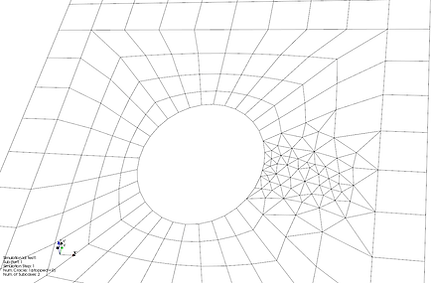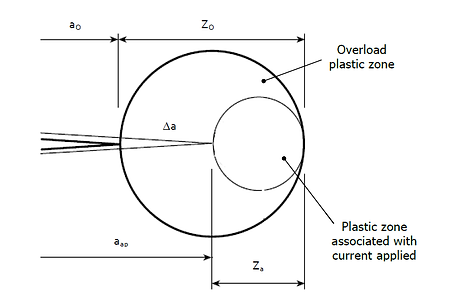###### GROWTH

GROWTH is the module for running Crack Growth analyses in 2D structures and 3D solid sections.
By means of this module, the following can be done in case of 2D structures.

• Running the analysis at a user defined FEM sub-model (provided this is a planar structure). An internal FEM solver handling plane-stress or plane strain elements is used.

• Calculating Stress Intensity Factors with J-Integral or displacement correlation methods.

• Propagating one or multiple introduced cracks (GROWTH remeshes the FEM automatically).

• Introduce holes or crack stop drill holes in the structure (and accounting of such modifications).

• Calculating crack growth Life based on the NASGRO crack growth mathematical model (without or with the Generalized Willemborg Retardation model), once the Stress Intensity Factor curves are calculated at the crack propagation phase.

When a FEM sub-part is selected for a crack propagation simulation, the program converts the model to the 2nd order.

Boundary conditions of the selected sub-part are defined by importing the FEM result file (the program reads displacements and grid point force balance at the automatically identified edges of the selected sub-part).

​The crack(s) can be introduced with the GUI in a simple manner. The user can control the crack size, orientation and FEM refinement. At the crack tip a rosette of 8 quarter point triangular elements is placed.

This allows the calculation of Stress Intensity Factors (KI and KII), using J-Integral approach, with high fidelity.

With a similar GUI interaction process, the analyst can introduce holes or stop drills.

Once the 'damaged' model is defined, the analysis is performed as follows.

• Two nodes are constrained (even if the sub-part is self balanced, for numerical reasons the FEM must be properly conditioned). Typically these are selected in a location sufficiently far from the crack propagation region.

• A reference condition is defined by importing load case related sequences and selecting the instant for which the internal stresses have to be considered.

• It is defined whether the propagation has to be 'straight' or whether the crack can deviate according to the calculated ratios KII/KI.

• It is defined whether plane stress or plane strain elements have to be used (the analyst must decide in advance, depending on the thickness and fracture toughness.

​​​​​​​​​​​​​​​​​​In case of multiple propagating cracks, the program detects the faster crack and propagates the other(s) by scaling the propagation increments on the basis of the Paris exponent (user defined).

The propagation simulation delivers a set of SIF curves (one curve for each crack, made of 'points' collected by considering the SIFs at each propagation steps).

The SIF curves are used then as main input for calculating Crack Growth Life.
Modification factors can be defined (such as additional SIFs or scaling factors defined at each propagation steps). This is particularly relevant in case the analyst wants to account residual stress conditions.
The NASGRO equation model is used. When LIFING is installed the NASGRO database file is installed. The Generalized Willemborg Retardation model can be used.

The SIF curves are used then as main input for calculating Crack Growth Life.
Modification factors can be defined (such as additional SIFs or scaling factors defined at each propagation steps). This is particularly relevant in case the analyst wants to account residual stress conditions.
The NASGRO equation model is used. When LIFING is installed the NASGRO database file is installed. The Generalized Willemborg Retardation model can be used.On the left, the GROWTH workflow is summarized:

1. The FEM is imported.

2. A sub-part of the model to be analysed for crack growth is defined.

3. The selected sub-part is opened in a dedicated dialog, where the user defines:

a. the boundary conditions

b. the crack(s)

c. the nature of propagation (straight or kinking)

4. The crack(s) is(are) propagated and related Stress Intensity Factor curve(s) is(are) calculated

5. The calculated SIFs are used with the NASGRO model and the CG Life is obtained.

For 3D solid sections the following process applies:

• Import a solid mesh FEM and store all internal nodal stresses in the LIFING database

• Cut a section

• Introduce a crack (elliptical corner crack or surface crack)

• Run the crack growth analysis (AFGROW style)

LIFING calculates SIFs on the surface and on the thickness and propagates the crack based on the input stress sequence.

Based on the stress distribution in the section the crack shape changes while propagating.

The calculation of SIFs is based on the semi-empirical Newman-Raju solutions.

Stress distributions (in x and y directions) are accounted by means of Glinka's Weight Functions.The GROWTH module development Roadmap includes the following enhancements:

• Using Crack Growth da/dN curves given in tabular lookup format.

• Interaction with the LIFE module in order to calculate crack initiation at a hole which has been drilled inside the GROWTH module.